# The temp range

What is the range in temperatures if the high temperatures is 67 degrees Celsius and the low temperature is -25 degrees Celsius?

r =  92 °C

### Step-by-step explanation: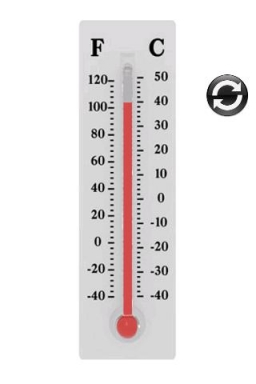Did you find an error or inaccuracy? Feel free to write us. Thank you!## Related math problems and questions:

• Change in temperatureStarting temperature is 21°C, the highest temperature is 32°C. What is the change in temperature?
• The lowestThe lowest temperature on Thursday was 20°C. The lowest temperature on Saturday was 12°C. What was the difference between the lowest temperatures?
• Difference in temperaturesThe temperature in the number line show minus 15 degree celsius and 8 degree celsius. What is the difference between the temperatures?
• The temperature 11The temperature on Sunday was 4°C. The temperature dropped 8°C on Monday and twice as much on Tuesday. What is the temperature on Tuesday?
• The temperature 10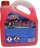The temperature in a freezer is -15°C, and it increases by 3°C.
• The temperature 3The temperature is -12 degrees Fahrenheit. During the following 5 hours, the temperature decrease by 23 degrees Fahrenheit. What is the weather at 11 pm?
• Now isNow is 5°C morning. What will the temperature be if i. It rises by 12°C ii. It falls by 15°C
• Difference of temperaturesThe temperature on Saturday was –2°C. The temperature on Sunday was 8°C. Write down the difference between these two temperatures.
• Mixing Celsius and FahrenheitAdd up three temperatures: 5°F +6°F +0°C
• Temperatures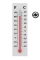Temperatures in degrees Fahrenheit and Celsius are related by the rule F = 1.8C + 32. What is the temperature in degrees Celsius if it is 50°F?
• 8 degrees8 degrees Celsius it cools down by 9 degrees Celsius. What is the resultant temperature?
• If the 2If the temperature is 86 degrees in Fahrenheit, what is the same temperature in Celsius?
• Celsius 25 degrees Celsius at midday dropped 12°Celsius degree by evening. What is the temperature?
• At midmorningThe temperature at midmorning was 31°C. Early in the evening, the temperature dropped by 5°C. What was the resulting temperature?
• The level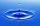The level of temperature of the liquid in a thermometer is 24.32°C lower than the boiling point of water. What is the thermometer reading? Show your answer by drawing and shading the thermometer.
• Temperature up and dowm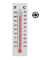At 6 AM, the temperature is 19.4°C. Between 6 AM and noon, the temperature rises 3.8°C. Between noon and 6 PM, the temperature falls 2.5°C. What is the temperature at 6 PM?
• WeatherWeather. At 5:00 am, the temperature was 28°C, and at noontime, it rose by 9°C. By 4:00 pm, it dropped 3°C, and by nighttime, it was 4°C less. What is the room temperature this time?